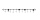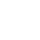# Fractions + mixed numbers - math problems

#### Number of problems found: 67

• Mixed numbersRewrite mixed numbers, so the fractions have the same denominator: 5 1/5 - 2 2/3
• Addition of mixed numeralsAdd two mixed fractions: 2 4/6 + 1 3/6
• Difference mixed fractionsWhat is the difference between 4 2/3 and 3 1/6?
• Between two mixedWhat is the rational number between 2 1/4 and 2 4/5?
• Mixed divided with fracThere are 2 2/3 pizzas. How many people are sharing when each has 2/3 of pizza?
• Adding mixed numbersAdd this two mixed numbers: 1 5/6 + 2 2/11=
• Mixed2improperWrite the mixed number as an improper fraction. 166 2/3
• Mixed with percentagesCalculate 33 1/3% of 570.
• Divide 11Divide the product of 4 and 5/8 by 1 1/2. Write your answer as a mixed number.
• Add sub fractionsWhat is 4 1/2+2/7-213/14?
• To improper fractionChange mixed number to improper fraction a) 1 2/15 b) -2 15/17
• Equivalent fractions 2Write the equivalent multiplication expression. 2 1/6÷3/4
• Equation with mixed fractions2 3/5 of 1430+? = 1900. How to do this problem
• Free timeAnita had 2 1/4 hours of free time in the afternoon. She spent 2/3 of her free time playing sports and watched TV for 30 minutes. How much time did she have left to read the book? Express the result in fractions in hours and numbers in minutes.
• Cubic inches to cups5-3/8" x 4" x 2-5/8" container. How many equals cups?
• Adding mixed 3Why does 1 3/4 + 2 9/10 equal 4.65? How do you solve this?
• Clock mathematicsIf it is now 7:38 pm, what time will it be in 30,033,996,480 minutes from now?
• Adding mixed 42 and 1 8th plus 1 and 1 3rd =
• Two pizzasJacobs mom bought two whole pizzas. He ate 2/10 of the pizza and his dad ate 1 1/5. How much is left.
• An airplaneAn airplane flies 1440 km in 2 1/4 hours. What is its average speed in km per hour?

Do you have an interesting mathematical word problem that you can't solve it? Submit a math problem, and we can try to solve it.

We will send a solution to your e-mail address. Solved examples are also published here. Please enter the e-mail correctly and check whether you don't have a full mailbox.

Please do not submit problems from current active competitions such as Mathematical Olympiad, correspondence seminars etc...

Need help to calculate sum, simplify or multiply fractions? Try our fraction calculator. Mixed numbers - math problems. Fraction Word Problems.